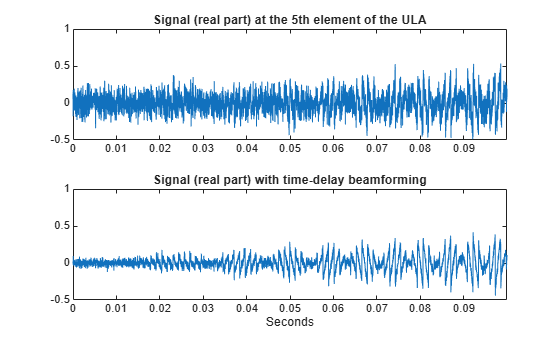# Time-Delay Beamforming of Microphone ULA Array

This example shows how to perform wideband conventional time-delay beamforming with a microphone array of omnidirectional elements. Create an acoustic (pressure wave) chirp signal. The chirp signal has a bandwidth of 1 kHz and propagates at a speed of 340 m/s at ground level.

Note: This example runs only in R2016b or later. If you are using an earlier release, replace each call to the function with the equivalent `step` syntax. For example, replace `myObject(x)` with `step(myObject,x)`.

```c = 340; t = linspace(0,1,50e3)'; sig = chirp(t,0,1,1000);```

Collect the acoustic chirp with a ten-element ULA. Use omnidirectional microphone elements spaced less than one-half the wavelength at the 50 kHz sampling frequency. The chirp is incident on the ULA with an angle of $6{0}^{\circ }$ azimuth and ${0}^{\circ }$ elevation. Add random noise to the signal.

```microphone = phased.OmnidirectionalMicrophoneElement(... 'FrequencyRange',[20 20e3]); array = phased.ULA('Element',microphone,'NumElements',10,... 'ElementSpacing',0.01); collector = phased.WidebandCollector('Sensor',array,'SampleRate',5e4,... 'PropagationSpeed',c,'ModulatedInput',false); sigang = [60;0]; rsig = collector(sig,sigang); rsig = rsig + 0.1*randn(size(rsig));```

Apply a wideband conventional time-delay beamformer to improve the SNR of the received signal.

```beamformer = phased.TimeDelayBeamformer('SensorArray',array,... 'SampleRate',5e4,'PropagationSpeed',c,'Direction',sigang); y = beamformer(rsig); subplot(2,1,1) plot(t(1:5000),real(rsig(1:5e3,5))) axis([0,t(5000),-0.5,1]) title('Signal (real part) at the 5th element of the ULA') subplot(2,1,2) plot(t(1:5000),real(y(1:5e3))) axis([0,t(5000),-0.5,1]) title('Signal (real part) with time-delay beamforming') xlabel('Seconds')```